•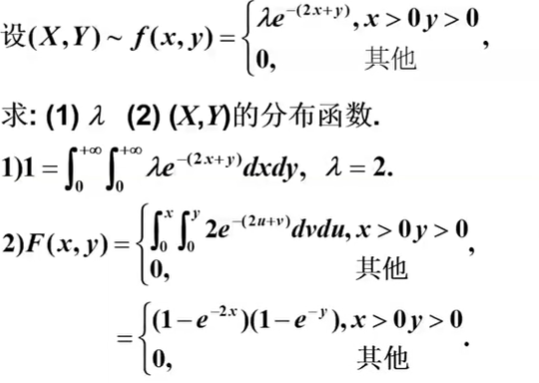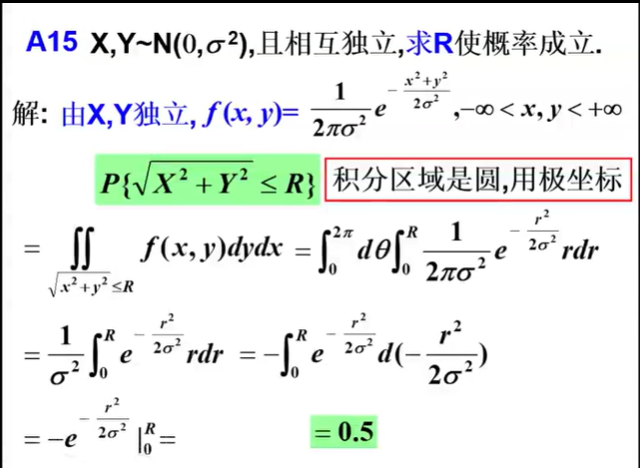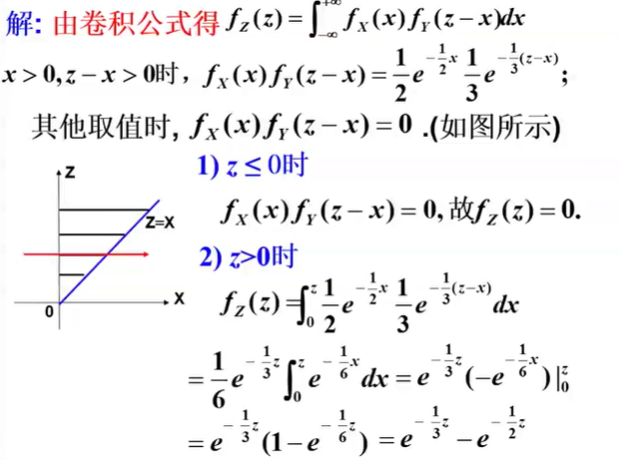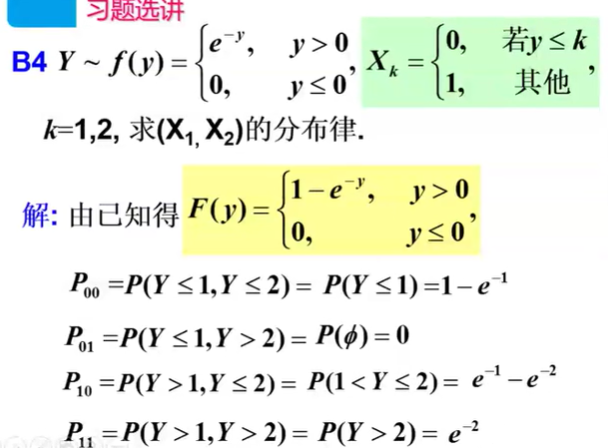展开全文• 在概率论中学到的二维随机变量的协方差公式到底对应什么物理意义呢，为什么就能度量两个随机变量之间之间相关性了呢？这里带着大家，结合随机变量取值图像来实际理解这个公式，从此以后不再是死记硬背，而是通熟易懂...
在概率论中学到的二维随机变量的协方差公式到底对应什么物理意义呢，为什么就能度量两个随机变量之间之间相关性了呢？这里带着大家，结合随机变量取值图像来实际理解这个公式，从此以后不再是死记硬背，而是通熟易懂的理解了它的实际意义我们就能更好地使用它，这个在统计学，机器学习等方面都有运用。文章简短，一看就会！！！
先上公式：
协方差：Cov(X,Y) = E[(X-E[X]) (Y-E[Y])]
相关系数：r(X,Y) = Cov(X,Y) / ( sqrt(Var[X]) * sqrt(Var[Y] )     注：Var[X]代表随机变量X的方差

从相关系数公式可以看出，相关系数公式只是把 协方差算出来的值 除以了 两个方差的开根号值，也就是相关系数跟协方差是一种正比例关系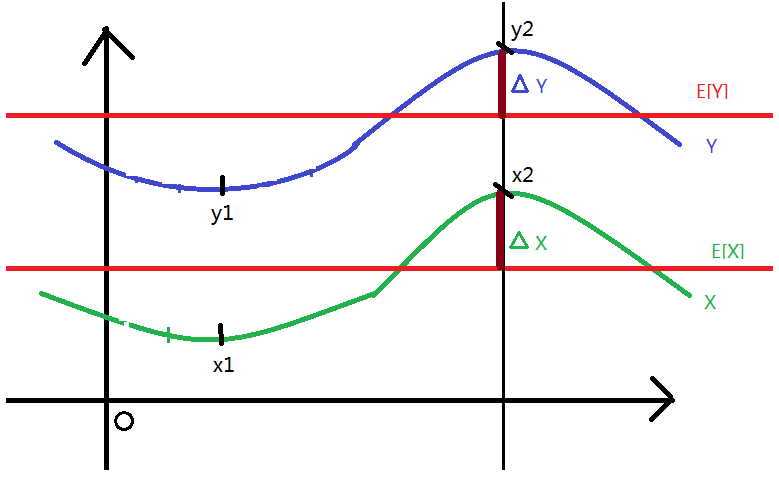注：图中分别有两个随机变量X,Y，其中x1,x2.....xn 是X变量的所有取值，y1,y2.....yn 是Y变量的所有取值
红色的线代表自己的均值，▲X代表X变量当前取值和自己均值的差，▲Y代表X变量当前取值和自己均值的差

从相关这个词语的意思可以知道，如果两个变量X,Y相关性越大，那么他两的取值方式（都同时取了均值之上呢还是相反呢）应该越像：如上图所示
比如X变量当前取值x1是小于自己的均值E[X]的（▲x1 = x1-E[X] <0），同时Y当前取值y1也是小于自己的均值E[Y]的      （▲y1 = y1-E[X] <0），那我们就说X,Y当前的的取值方式一样，x1,y1两点正相关
比如X变量当前取值x2是大于自己的均值E[X]的（▲x2 = x2-E[X] >0），同时Y当前取值y2也是大于自己的均值E[Y]的         （▲y2 = y2-E[X] >0），那我们还是说X,Y当前的取值x2,y2正相关，因为取值方式确实还是一样的，都是在均值之上。
正相关判定性：上述两个式子等价于这个式子▲X*▲Y=（xi-E[X]）* （yi-E[Y]）>0
很明显上面图中X,Y两个随机变量所有的取值应该是正相关的，因为X,Y两个随机变量取值与均值差的正负性完全相同，那么总体上肯定就是正相关。就是上下波动性相同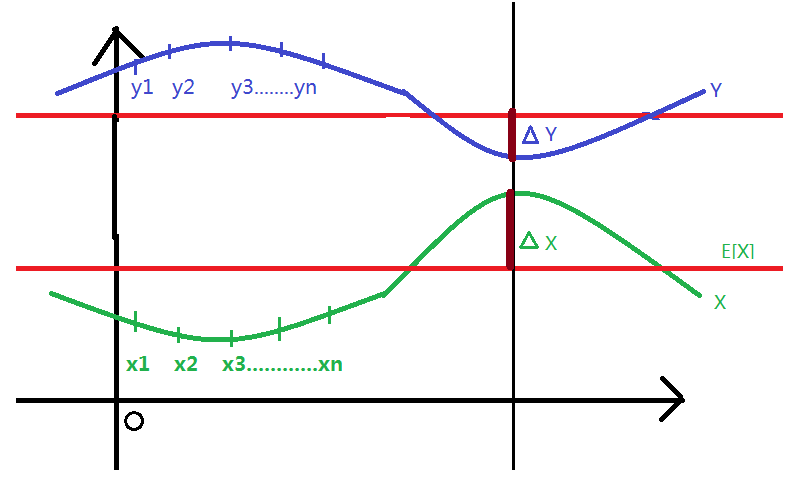既然我们知道如何判断正相关，同理，判断负相关就是，两者的取值方式是否刚好相反，
比如上图所示：xi取值在均值下方，此时的yi在均值上方，那我们就说他们取值方式相反，即负相关
或者 xi取值在均值上方，此时的yi在均值下方，那我们就说他们取值方式相反，也是负相关
负相关判定性：就等价于这个式子▲X*▲Y=（xi-E[X]）* （yi-E[Y]）<0
很明显上面图中X,Y两个随机变量所有的取值应该是负相关的，因为X,Y两个随机变量取值与均值差的正负性完全相反，那么总体上肯定就是负相关。也就是波动性相反
由于随机变量X,Y可以取很多个可能的值，上面我们已经得到了每个点的正负相关性，但是我们需要分析X,Y的整体相关性，所以我们需要把他们每个点的相关性都累加起来，然后取个平均值，就可以知道X,Y的整体相关性了
也就是：( ((x1-E[X])*y1-E[Y]) +  ((x2-E[X])*y2-E[Y]) +........((xn-E[X])*(yn-E[Y]) ) / n          （1）
其中x1,x2.....xn 是X变量的可能取值，E[X]是X变量的均值，即E[X] = (x1+x2+...xn) / n
其中y1,y2.....yn 是Y变量的可能取值，E[Y]是X变量的均值，即E[Y] = (y1+y2+...yn) / n

根据 均值 E[Z] = (z1+z2+z3+.....zn) / n 的定义公式，上面这个公式（1）不就是 E[(X-E[X]) (Y-E[Y])] 嘛，这个就是协方差的公式呀，所以协方差就是度量了X,Y两变量的整体相关性，即X,Y取值的方式相似性，都同时取了均值之上呢还是相反呢。
然后 Cov(X,Y) / ( sqrt(Var[X]) * sqrt(Var[Y] )，就是把协方差除以两个变量各自的方差（方差是常数），
这样操作后，可以理解为使得相关系数就是协方差被归一化了为【0，1】之间取值了（有兴趣自己去测试一下），更便于我们进行各种计算。

上面两图，如果题目给出了具体的值x1,x2...xn,y1.y2...yn，我们就可以进行计算相关系数了，
上面第一个图因为两变量取值方式完全相同，即完全正相关，相关系数算出来肯定=1
上面第二个图因为两变量取值方式完全相反，即完全负相关，相关系数算出来肯定=-1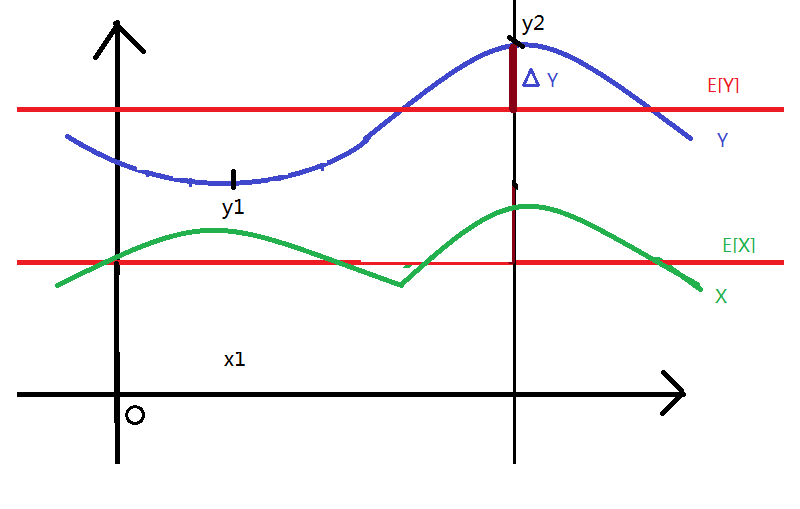如上图所示：如果左边一部分取值负相关，右边一部分正相关，也就是左边X和Y在均值上下取刚好相反，右边部分的同时在均值之上，总体算出来，X,Y相关系数可能是0（即不相关），也可能是0.2等，也可能是-0.3等等，即相关性没那么强。

总结：所以，协方差是两个变量X,Y相关性的一种度量，而相关系数就是协方差归一化的表现，
协方差正值越大代表正相关性越强，即X,Y同时取均值之上或者之下的值更多，整体来看，X,Y上下波动性（图像走势）同向（不太严谨，但是便于通俗理解）。
协方差负值越大代表负相关性越强，即X,Y一个取均值之上，同时另一个取均值之下的值更多（图像走势相反）。
如果协方差为0，代表不相关，整体来看，X,Y取值方式相反的点相互抵消了，即一部分上下波动同向，一部分反向，总体就相互抵消了
因此，相关性就是度量了X,Y两变量的整体相关性，即X,Y取值的方式相似性，都同时取了均值之上呢还是相反呢。大概理解上来说，就是指随机变量X,Y取值的上下波动方向性（走势）相同的程度
展开全文协方差
• 概率论对于学习 NLP 方向的人，重要不言而喻。于是我打算从概率论基础篇开始复习，也... - 概率密度 边缘概率密度 条件概率密度 独立 需要有二重积分相关知识 - Z = X + Y 分布 Z = XY 分布 Z = max{X, Y} 分布

概率论对于学习 NLP 方向的人，重要性不言而喻。于是我打算从概率论基础篇开始复习，也顺便巩固巩固基础。 这是基础篇的第六篇知识点总结 注意：复杂的公式例如卷积公式等将在概率论高阶中提到

基础：下面前五篇的链接地址： 概率论基础（1）古典和几何概型及事件运算 概率论基础（2）条件概率、全概率公式和贝叶斯公式 概率论基础（3）一维随机变量（离散型和连续型） 概率论基础（4）五种重要的分布（二项、泊松、均匀、指数、正态分布） 概率论基础（5）离散型二维随机变量
连续型二维随机变量
知识点
概率密度边缘概率密度条件概率密度独立性需要有二重积分相关知识
定义可自行查询
常用性质： 根据前面几节的回顾，加上了解其定义，不难理解有以下性质：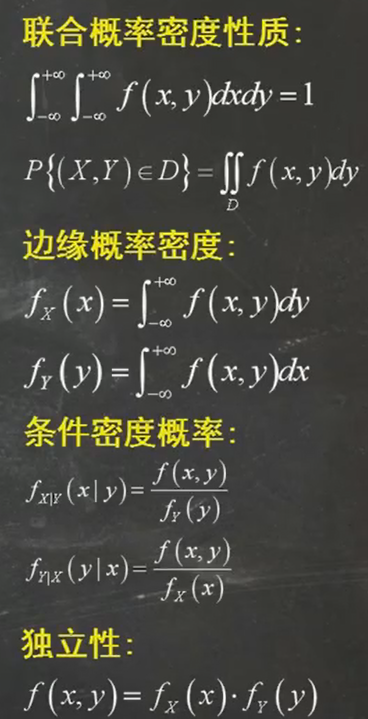例题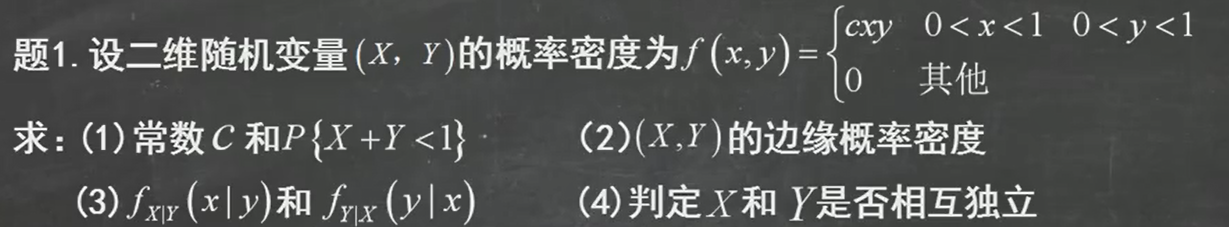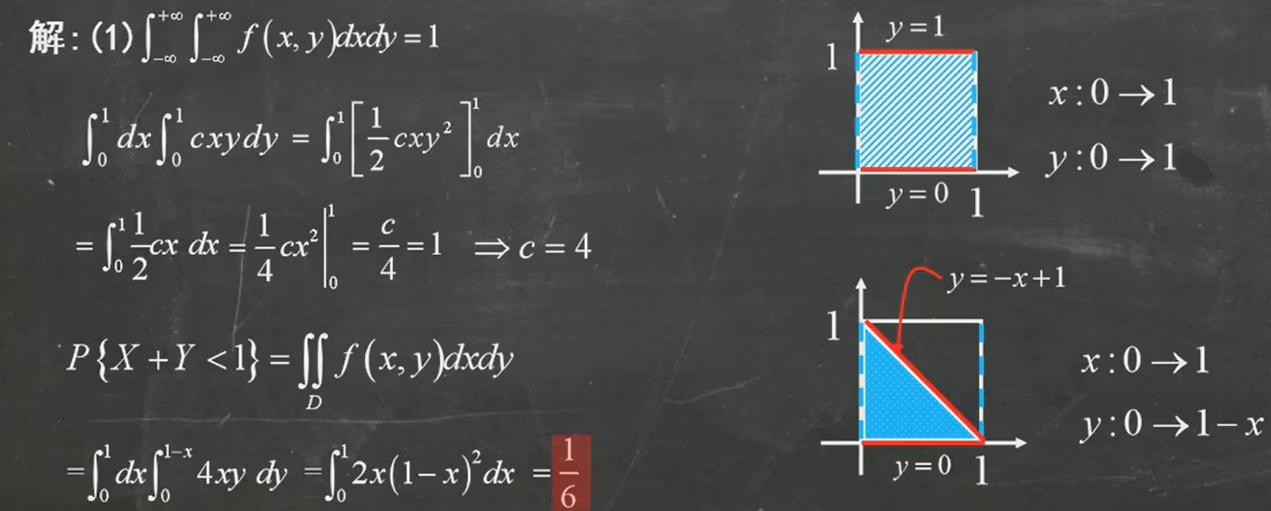理解：在这里用到了第一个公式性质，运用二重积分的计算，可直接求出 c 的值为4。求出 c 后，要求解P（X+Y<1）重点是分析D的取值范围，如果较为熟悉二重积分，很快就能确定其范围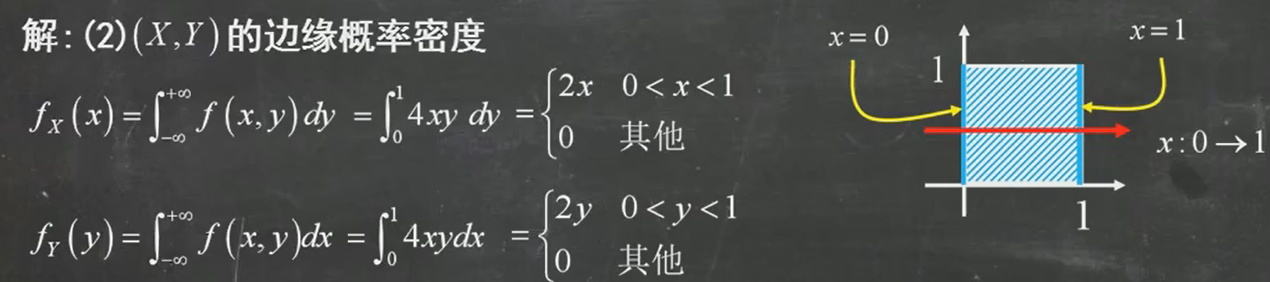理解：求x的边缘概率密度，其实就是对y进行求偏微分，反之亦然。
注意：写概率密度的形式，一般是大括号，然后标清区间的位置，其他为0.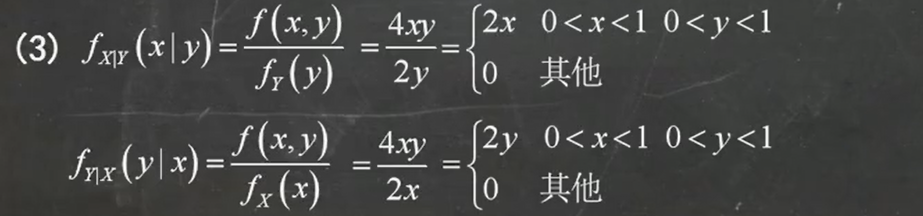理解：注意条件概率密度的计算公式即可，根据前面求的，直接代入。理解：根据最后一条性质，根据其式子直接判断即可。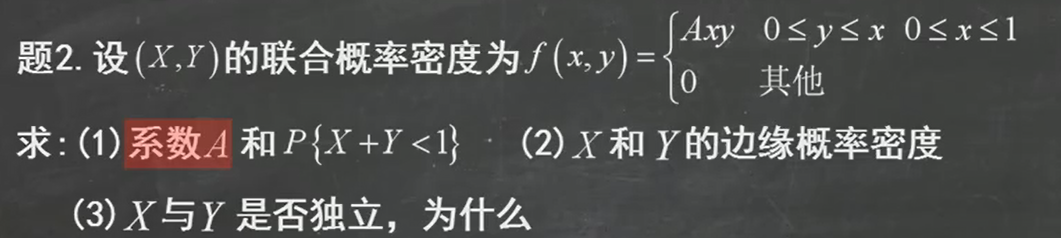注意：它的区间不再是简单的孤立，而是相互关联起来。
仿照上述题目，即可解决问题：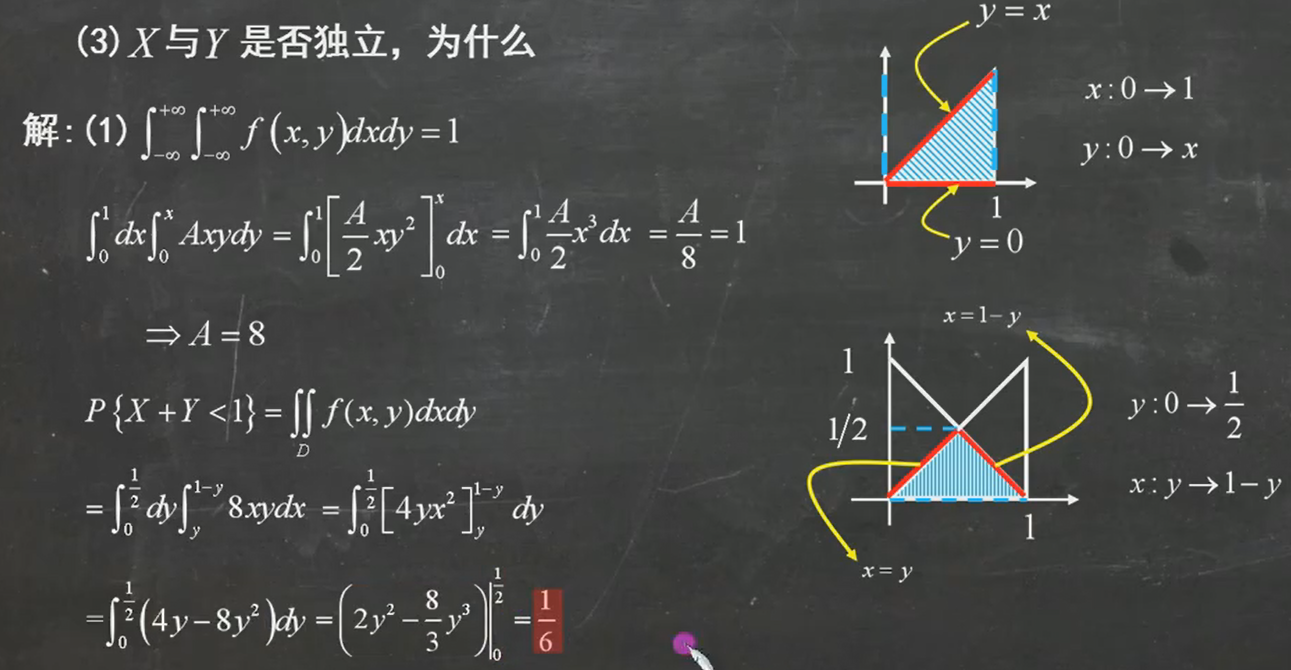注意：当从x的范围到y不好分析时，可以利用y来求解x的范围，注意一下求解技巧。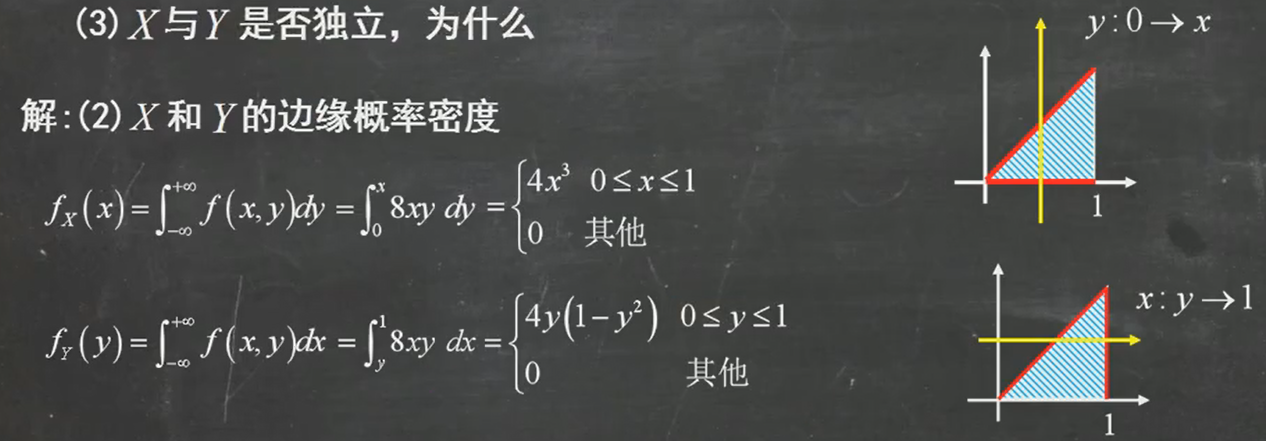理解：与上题1类似。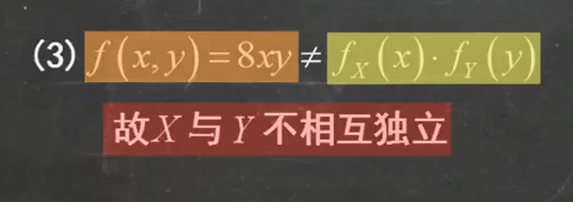理解：可以直接利用第二问求解的值，来判断。
放一点额外的练习题，来巩固基础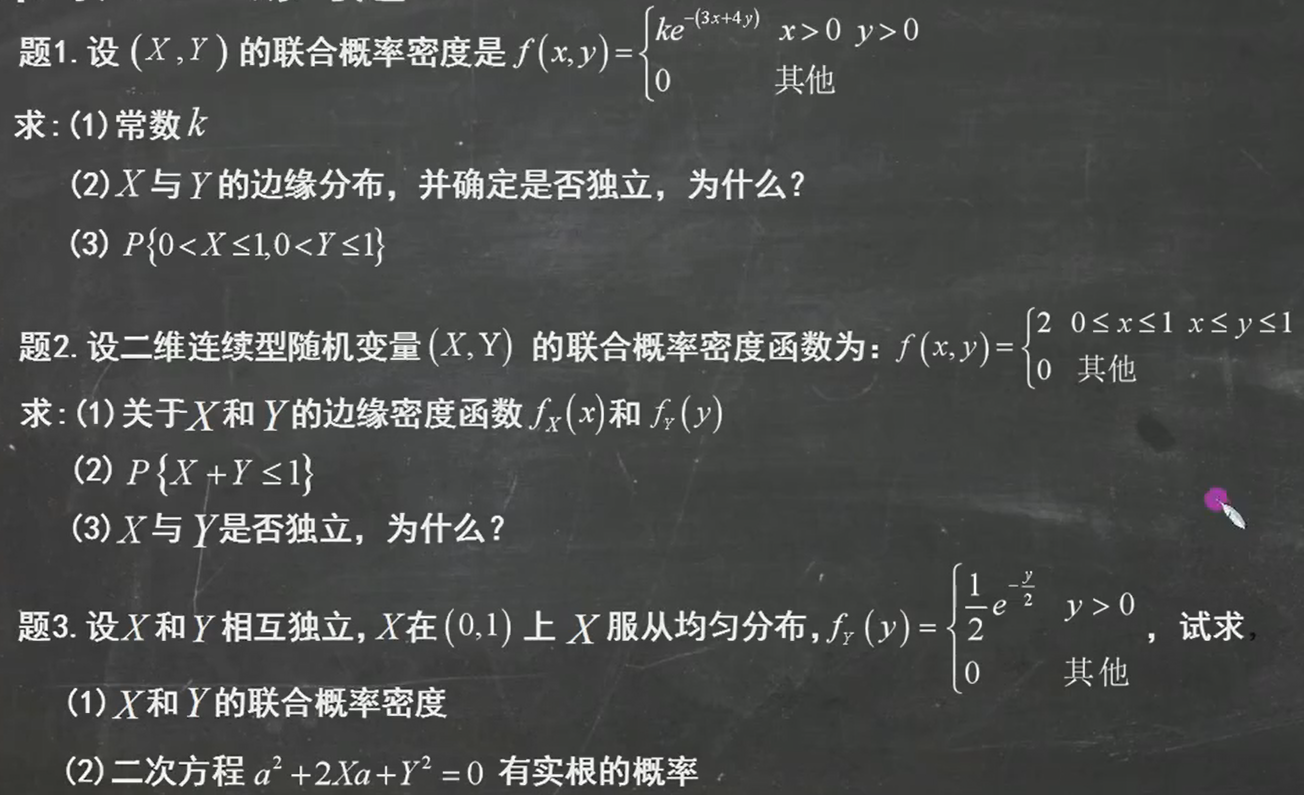连续型二维随机变量函数的分布
知识点
Z = X + Y 分布Z = XY 分布Z = max{X, Y} 分布
Z = X + Y 分布 它有一个常用的解题步骤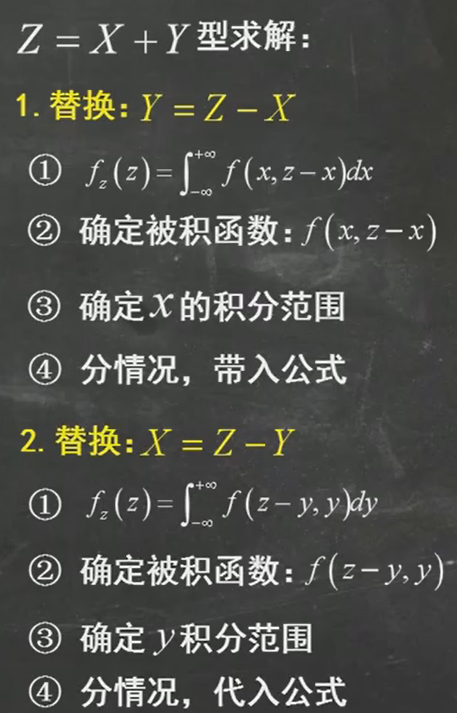具体选择哪一种的依据：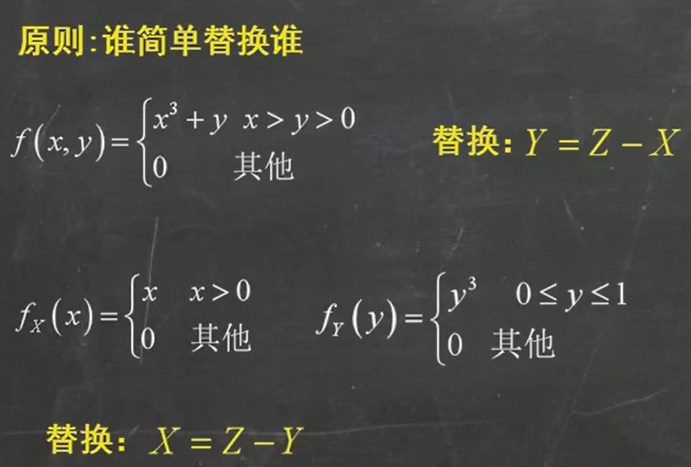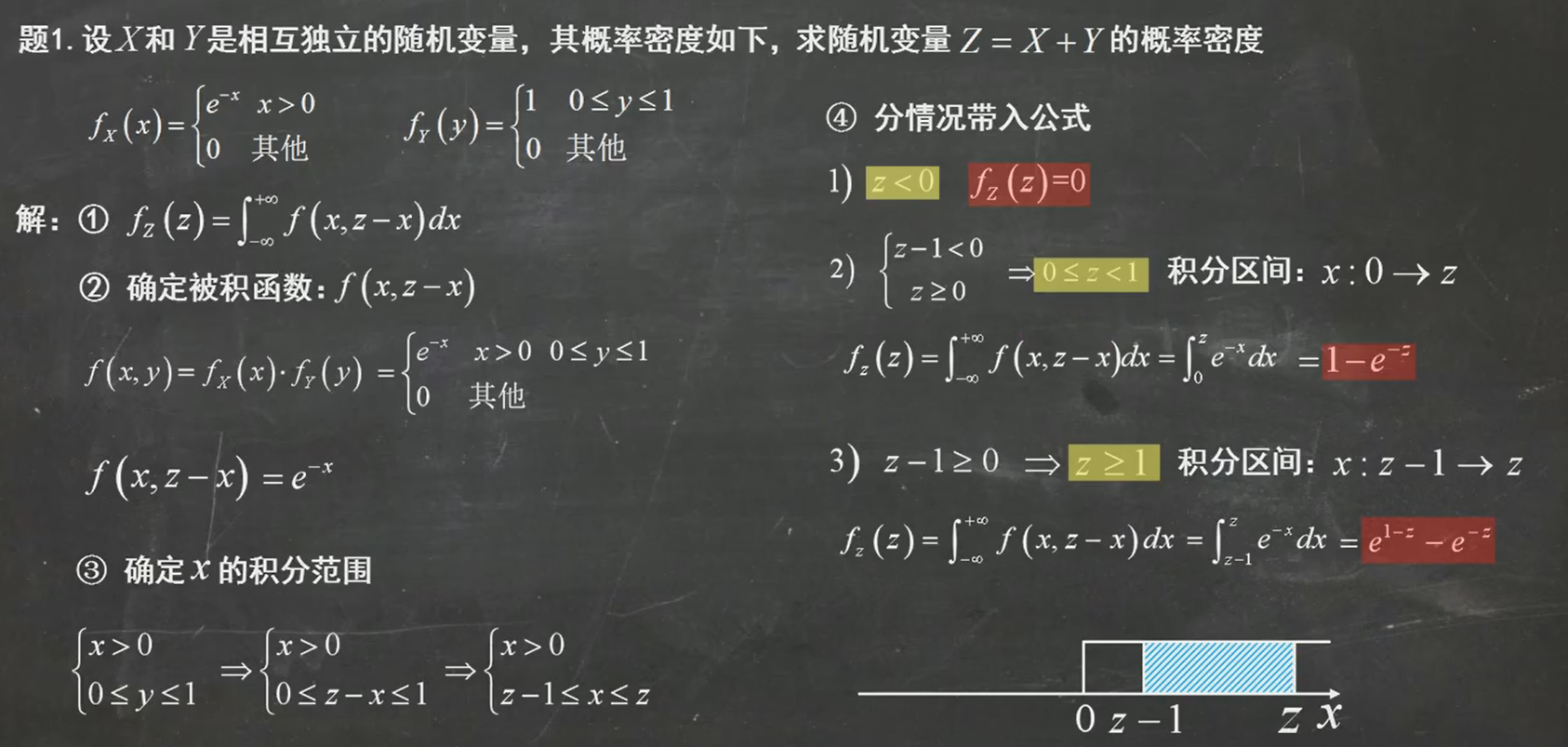注意：可以画图来分析Z在坐标轴上的分布情况，从而分类处理 最终结果：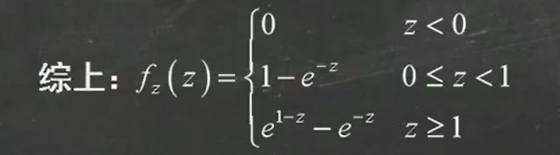Z = XY 分布 这种分布也有常用的解题方法步骤：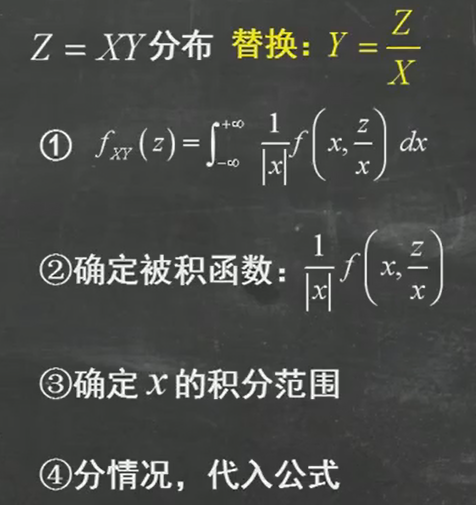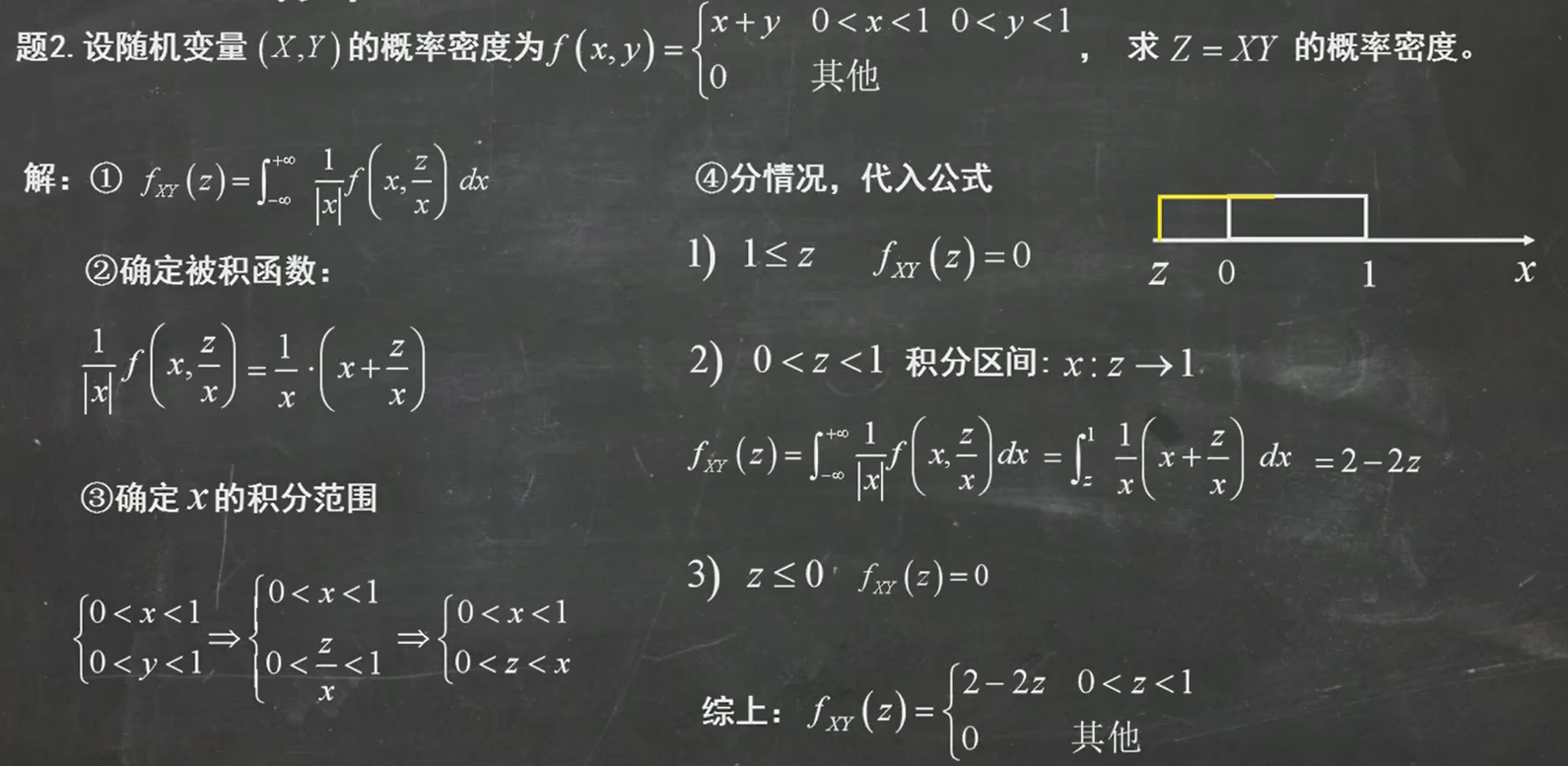理解：需要注意的是，当z <= 0 时，由于z的范围是0 < z < x ，因此它是没有意义的，所以取0
Z = man{X, Y}的分布 它的常用解题步骤：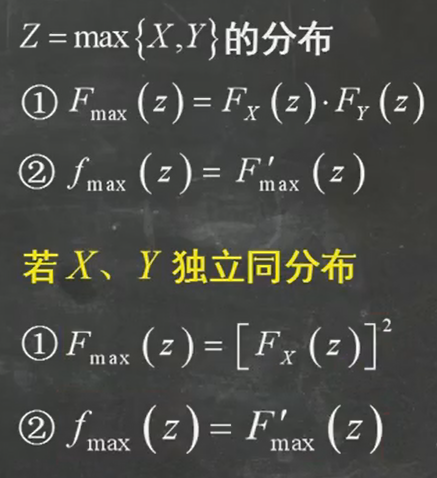例题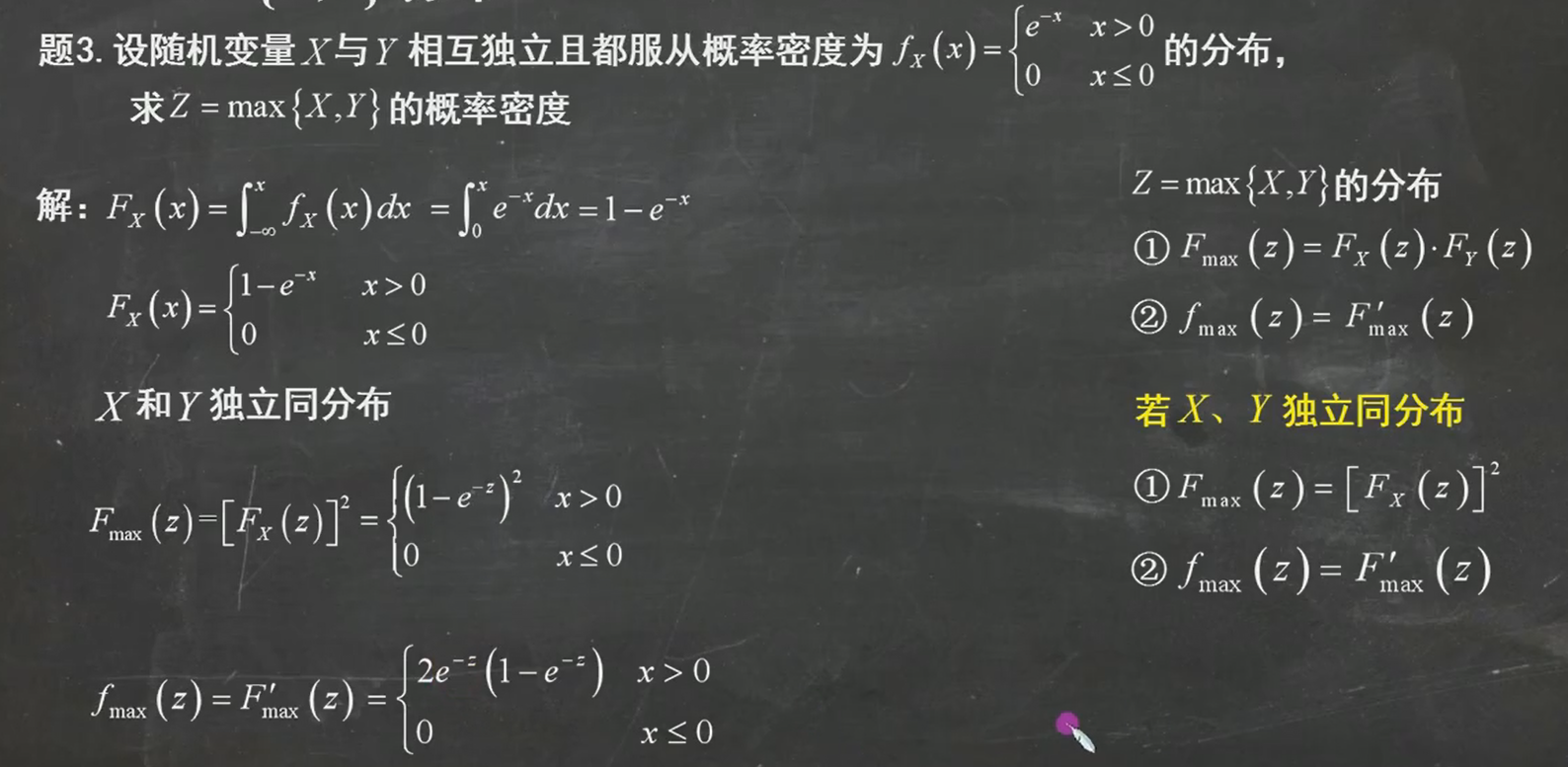理解：先用公式解出z的分布函数，其概率密度就是其分布函数的求导结果
这里给两个常用的结论：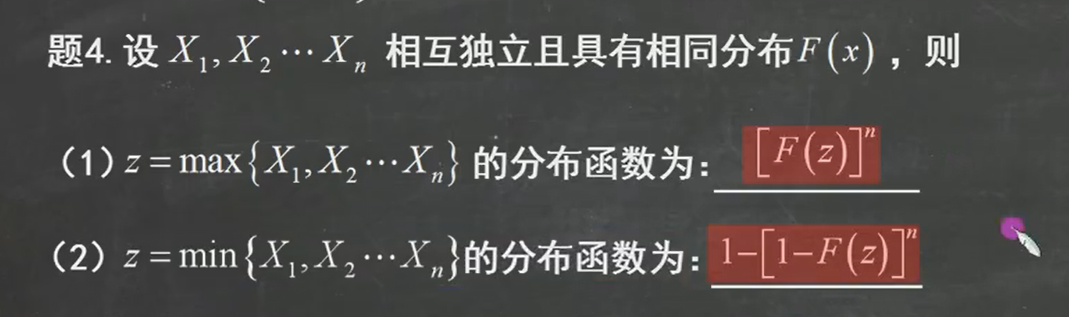额外放一点练习题：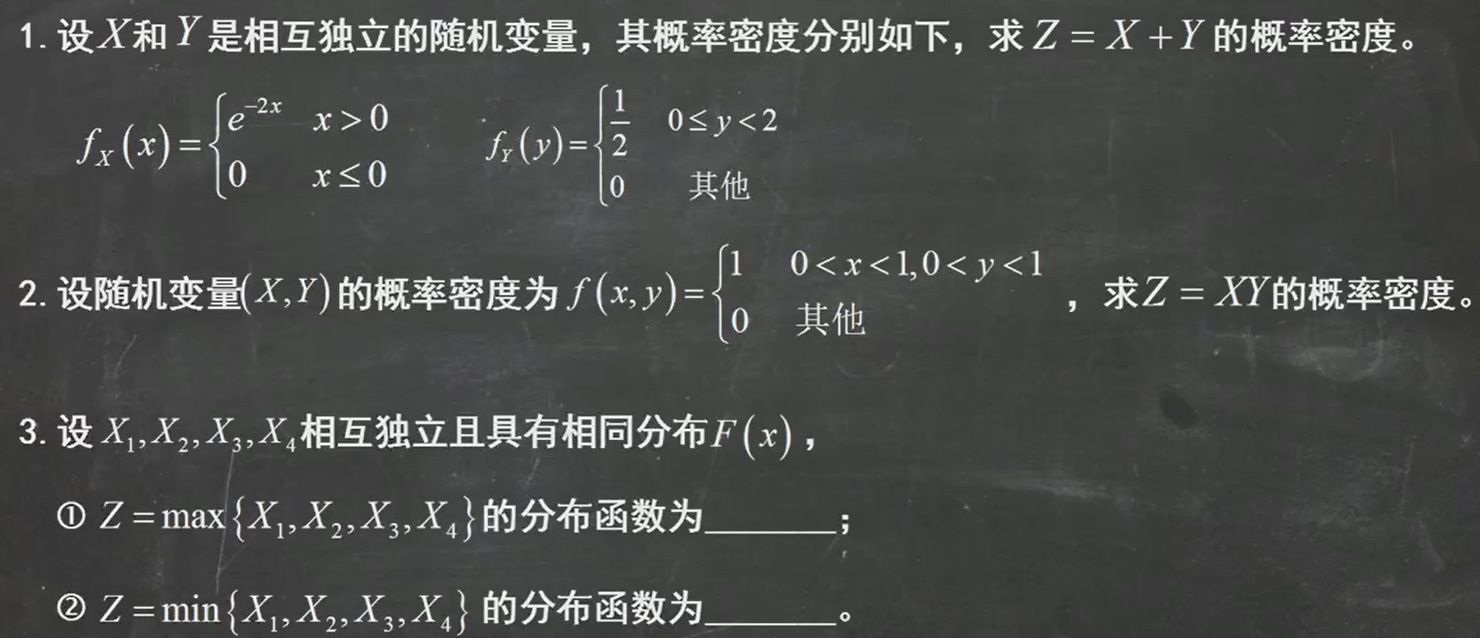展开全文NLP基础
• 二维随机变量期望的计算@(概率论)设随机向量(X,Y)的概率密度f(x,y)满足f(x,y) = f(-x,y),且ρxy\rho_{xy}存在，则ρxy=?\rho_{xy} = ?分析：主要从EXY, EX,EY的关系求解。 因为根据定义：ρxy=cov(X,Y)DX√DX√\rho...
二维随机变量期望的计算
@(概率论)
设随机向量(X,Y)的概率密度f(x,y)满足f(x,y) = f(-x,y),且

ρxy
$\rho_{xy}$存在，则

ρxy=?
$\rho_{xy} = ?$
分析：主要从EXY, EX,EY的关系求解。  因为根据定义：

ρxy=cov(X,Y)DX√DX√
$\rho_{xy} = {cov(X,Y)\over \sqrt {DX}\sqrt{DX}}$
而

cov(X,Y)=E(XY)−EXEY
$cov(X,Y) = E(XY) - EXEY$
这些是最基础的特征。
再看

EXY
$EXY$的求法：

EXY=∫+∞−∞∫+∞−∞xyf(x,y)dxdy
$EXY = \int_{-\infty}^{+\infty} \int_{-\infty}^{+\infty}xyf(x,y)dxdy$
更值得注意的是，EX，EY的求法：

EX=∫+∞−∞∫+∞−∞xf(x,y)dxdy
$EX = \int_{-\infty}^{+\infty} \int_{-\infty}^{+\infty}xf(x,y)dxdy$
也将x看成是g(X,Y)，对Z = g(X,Y)求期望，自然是统一的二次积分。
所以，

EXY=∫+∞−∞∫+∞−∞xyf(x,y)dxdy=∫+∞−∞ydy∫+∞−∞xf(x,y)dx=∫+∞−∞ydy∫−∞+∞−tf(−t,y)d(−t)[令x=−t]=∫+∞−∞ydy∫−∞+∞tf(t,y)dt=0[因为左右相反却相等]

EXY = \int_{-\infty}^{+\infty} \int_{-\infty}^{+\infty}xyf(x,y)dxdy \\
= \int_{-\infty}^{+\infty} ydy\int_{-\infty}^{+\infty}xf(x,y)dx \\
= \int_{-\infty}^{+\infty} ydy\int_{+\infty}^{-\infty}-tf(-t,y)d(-t) [令x=-t]\\
= \int_{-\infty}^{+\infty} ydy\int_{+\infty}^{-\infty}tf(t,y)dt  = 0[因为左右相反却相等]

同理，EX= 0也是一样的原因，所以EXY = EXEY = 0,所以相关系数为0。
展开全文• 二维随机变量（向量） 设EEE是一个随机试验，它的样本空间是S=eS={e}S=e,设X=X(e)X=X(e)X=X(e)和Y=Y(e)Y=Y(e)Y=Y(e)是定义在SSS上的随机变量，由它们构成的一个向量(X,Y)(X,Y)(X,Y)叫做二维随机向量。 分布函数： ...
• 二维离散型随机变量独立定义的基础上,得到二维离散型随机变量独立的一个新的判断定理,并给出相关的应用 。
• 文章目录关于连续型二维随机变量概率密度函数边缘概率密度条件概率密度独立来做点练习题吧！ 关于连续型二维随机变量 概率密度函数 如果说对于定积分 ∫f(x)dx\int f(x) dx∫f(x)dx ，它所表示的含义如果说是求...
• 文章目录一、二维离散型随机变量函数的分布二、二维连续型随机变量函数的分布和分布密度 一、二维离散型随机变量函数的分布 二、二维连续型随机变量函数的分布和分布密度 首先，我们有两个随机变量 X, Y。它们的概率...
• 二维正态随机变量是最常见的一种二维随机变量分布。其联合概率密度函数为： p(x,y)=12πσXσY1−r2⋅exp{−12(1−r2)[(x−mX2)σX2−2r(x−mX)(y−mY)σXσY+(y−mY2)σY2]} p(x,y)=\frac{1}{2\pi \sigma _X\sigma ...
• 二维随机变量 联合分布函数 定义 性质 边缘分布函数 联合密度 边缘密度 期望 方差
• 离散型随机变量的分布2.1 概率函数与分布函数定义2.2 分布函数的性质2.3 几种常见的分布1、项分布，记为$X \sim F$2、泊松分布，记为$X \sim P(\lambda)$3、超几何分布4、负项分布4. 连续型随机变量的分布4.1 ...
• 重要公式回顾 排列：从n个不同元素中取出m个元素排成一列的可能情况有 A(n,m种。 组合：从n个不同元素中...概率论中方差用来度量随机变量和其数学期望（即均值）之间的偏离程度。 统计中的方差（样本方差）是各个
• 使用多种方法实现二维离散随机变量概率分布的计算. 可以用于计算互信息等.hist3 accumarray mex
• 对于n维度空间中的点,若是n维随机变量，也就说记录的每一个字段都是随机变量，然后组成一个由随机变量组成的向量，称为随机向量 ，它的分布函数定义为 , 若 非负可积函数实值函数使得 那么称为随机向量，概率密度...
• 费勒的《概率论及其应用》并未找到随机变量相关的定义 当然，如很多书籍一样，有相关系数的概念。针对两个正态分布的随机变量： 抄一段原话：使用相关系数，无非是给协方差的书写来个花样罢了。很不幸，相关系数...协方差
• 网上看到了很多关于随机变量“不相关”“独立”两个概念的辨析，其中很多人用如单位元来形象说明，但是大多解释并不清晰 附上 陈希孺《概率论和数理统计》中的解释 因此： （1）陈希孺书中有“不相关”定义，这...协方差
• 两个随机变量相关系数指的是线性相关系数。 转载于:https://www.cnblogs.com/kevinGaoblog/archive/2012/05/19/2508937.html
• X和Y的（线性）相关系数是0。（为什么呢？直观来说，因为是个圆，如果你画一条线性回归的线，线的斜率是正的还是负的都不合适，因为是对称的。数学上） 因为 E(X|Y) = E(Y|X) = 0 所以 E(X) = E(Y) = 0，而且 E(XY) ...
• 切比雪夫不等式二、二维随机变量的数字特征1. 数学期望2. 协方差与相关系数（1）概念（2） 性质三、独立与相关性的判定 一、一维随机变量的数字特征 1. 数学期望 （1）概念定义 如果 XXX 是离散型随机变量，其...协方差
• 戳这里：概率论思维导图 ！！！ 数学期望 离散型随机变量的数学期望 （这里要求级数绝对收敛，若不绝对收敛，...二维随机变量的数学期望 （1）设(X,Y)是离散型随机变量，联合分布率为： 若绝对收敛，则Z=g(X,Y...
• 和其它问题一样，概率也可能同时受到多个条件的影响，例如考察某地区中学生的...本章以二维随机变量为例，介绍多维随机变量的相关概念。 联合分布 　和一维变量的概率分布类似，联合分布把舞台扩展到了多维，这里...
• 本文生成正态分布变量部分代码摘自《Random Signal Processing》，Shaila Dinkar Apte著，Taylor Francis Group出版。 正态分布 老办法实现： sigma=1; mean=0; x=(-10:0.1:10); a=(x-mean).*(x-mean); y=(1/(sigma*...matlab 统计学
• 一，二维随机变量及其分布函数 （1）二维随机变量 ：设随机变量 Z，X，Y；则有 Z{ X，Y }，一个随机变量是有两个随机变量决定的； （2）联合分布函数的基本性质： 单调：F（x，y）分别对x 或y是单调不减的...
• 文章目录前言数学期望与方差一维随机变量的期望和方差离散型连续型随机变量函数的期望离散型连续型推论运算性质常用随机变量的期望和方差协方差与相关系数 前言 说明 第一篇博客，主要内容是自己在学习概率论时的...
• 第二个问题：如何理解二维随机变量分布函数？分布函数对应的就是一个求概率的问题 为什么x>(-∞),y<(+∞)呢？？？脑补出坐标系就知道了，这表示的就是坐标系的范围。 单独取X或Y的分布函数称为关于X或Y的边缘分布...
• 二维正态分布中，参数ρ\rhoρ被定义为随机变量X,YX,YX,Y的相关系数，即ρ=r(x,y)\rho=r(x,y)ρ=r(x,y)。由 r(x,y)=Cov(x,y)Var[x]Var[y]Cov(x,y)=E[XY]−E[X]E[Y] r(x,y)=\frac{Cov(x,y)}{\sqrt{Var[x]Var[y]}} \...
• 1. 和的期望和方差两随机变量 x,z 统计独立，证明下列两个等式：
• 对任意两个随机变量X,Y，证明其相关系数的绝对值小于1。对任意两个随机变量X,Y，证明其相关系数的绝对值小于1。对任意两个随机变量X,Y，证明其相关系数的绝对值小于1。 证明思路： 先构造a+bX和Y的均方误差的期望E{...数学
• 概率与概率分布1.1离散型随机变量1.1.1概率质量函数1.1.2 累计分布密度函数1.1.3 Python的实现1.2 连续型随机变量2. 期望值与方差3.项分布4. 正态分布5. 其他连续分布5.1 卡方分布5.2 t分布5.3 F分布6. 变量的...python 统计模型 数据分析...